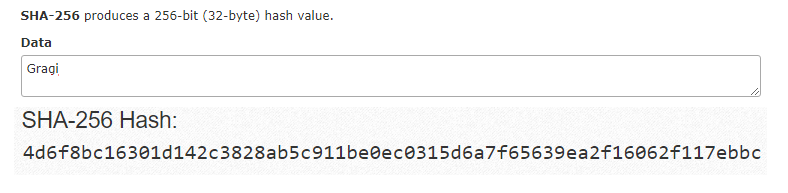Select Page

# Hashing in Blockchain

by | Jun 30, 2019 | Blockchain

Home » Blockchain » Hashing in Blockchain

Preface – This post is part of the Blockchain Basics series.

## Introduction

In the world of database, hashing is a very famous term. Either it is used to sort a table based on hash keys or to encrypt a data using hash function. In this article we will discuss only the second part that in encryption of data. This encrypted data is the actual transaction value stored in a block.

## What is Hashing

Hashing in blockchain is an encryption process in which an algorithm is used to convert a string of any length to a string of fixed length. It is a very important aspect in case of Blockchain as it helps us to keep track of huge transaction data without even saving them and rather saves hash of that data. There are various mechanism of hashing, such as Bitcoin uses SHA 256 while Ethereum uses Keccak-256 algorithms respectively. The only important thing to take away from this article is that this generated hash keys are the only that stores data of current block and address of the next block, hence helps in linking both the blocks and also keeping track of the previous block.

## How to do Hashing

If you have understood what a hashing is, then you will be thinking how to implement it. Different programing language provide different modules to hash a data. These modules take a data as input and returns fixed length output. The output length depends upon the algorithm used within the module. For example, if the algorithm is SHA-256, then the output length will be 256 bits long. You can find SHA-256 Module for Node.js here.

## Example of Hashing

In the diagram shown below, we have generated hash of a string using SHA 256 algorithm and you too can generate hash values from here. In ABAP you can use Method CALCULATE_HASH_FOR_RAW of Class CL_ABAP_MESSAGE_DIGEST to implement SHA-256 encryption hashing.SHA-256 Conversion

In the example shown below we will write a function in JavaScript to achieve SHA-256 encryption:

```var sha256 = function sha256(ascii) {
function rightRotate(value, amount) {
return (value>>>amount) | (value<<(32 - amount));
};

var mathPow = Math.pow;
var maxWord = mathPow(2, 32);
var lengthProperty = 'length'
var i, j; // Used as a counter across the whole file
var result = ''
var words = [];
var asciiBitLength = ascii[lengthProperty]*8;

//* caching results is optional - remove/add slash from front of this line to toggle
// Initial hash value: first 32 bits of the fractional parts of the square roots of the first 8 primes
// (we actually calculate the first 64, but extra values are just ignored)
var hash = sha256.h = sha256.h || [];
// Round constants: first 32 bits of the fractional parts of the cube roots of the first 64 primes
var k = sha256.k = sha256.k || [];
var primeCounter = k[lengthProperty];
/*/
var hash = [], k = [];
var primeCounter = 0;
//*/
var isComposite = {};
for (var candidate = 2; primeCounter < 64; candidate++) {
if (!isComposite[candidate]) {
for (i = 0; i < 313; i += candidate) {
isComposite[i] = candidate;
}
hash[primeCounter] = (mathPow(candidate, .5)*maxWord)|0;
k[primeCounter++] = (mathPow(candidate, 1/3)*maxWord)|0;
}
}

ascii += '\x80' // Append Ƈ' bit (plus zero padding)
while (ascii[lengthProperty]%64 - 56) ascii += '\x00' // More zero padding
for (i = 0; i < ascii[lengthProperty]; i++) {
j = ascii.charCodeAt(i);
if (j>>8) return; // ASCII check: only accept characters in range 0-255
words[i>>2] |= j << ((3 - i)%4)*8;
}
words[words[lengthProperty]] = ((asciiBitLength/maxWord)|0);
words[words[lengthProperty]] = (asciiBitLength)

// process each chunk
for (j = 0; j < words[lengthProperty];) {
var w = words.slice(j, j += 16); // The message is expanded into 64 words as part of the iteration
var oldHash = hash;
// This is now the undefinedworking hash", often labelled as variables a...g
// (we have to truncate as well, otherwise extra entries at the end accumulate
hash = hash.slice(0, 8);

for (i = 0; i < 64; i++) {
var i2 = i + j;
// Expand the message into 64 words
// Used below if
var w15 = w[i - 15], w2 = w[i - 2];
// Iterate
var a = hash, e = hash;
var temp1 = hash
+ (rightRotate(e, 6) ^ rightRotate(e, 11) ^ rightRotate(e, 25)) // S1
+ ((e&hash)^((~e)&hash)) // ch
+ k[i]
// Expand the message schedule if needed
+ (w[i] = (i < 16) ? w[i] : (
w[i - 16]
+ (rightRotate(w15, 7) ^ rightRotate(w15, 18) ^ (w15>>>3)) // s0
+ w[i - 7]
+ (rightRotate(w2, 17) ^ rightRotate(w2, 19) ^ (w2>>>10)) // s1
)|0
);
// This is only used once, so *could* be moved below, but it only saves 4 bytes and makes things unreadble
var temp2 = (rightRotate(a, 2) ^ rightRotate(a, 13) ^ rightRotate(a, 22)) // S0
+ ((a&hash)^(a&hash)^(hash&hash)); // maj

hash = [(temp1 + temp2)|0].concat(hash); // We don't bother trimming off the extra ones, they're harmless as long as we're truncating when we do the slice()
hash = (hash + temp1)|0;
}
```

## Author

•A Full Stack Developer with 10+ years of experience in technical content creation.

•